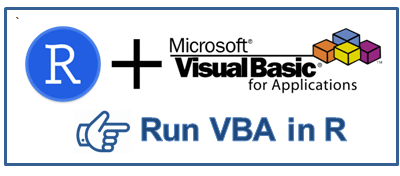# Run VBA in R

This tutorial describes how to run Visual Basic (VBA) script in R.VBA in R
Most of the times, we need to integrate R with Excel to make formating changes in excel workbook. With VBA, we can do a lot of things such as creating pivot table, applying functions, reshaping data, creating charts etc. It would help to automate the whole process. For example, building and validating a predictive model in R and exporting predictive probability scores to excel workbook. In R, call vb script to open the exported excel file and prepare gain and lift charts in excel.

Step 1 : Write VB Script and Save it as .vbs file

Sample Visual Basic Script

The following program tells Excel to open the workbook and apply borders to the used range in the sheet.
Set objExcel = CreateObject("Excel.Application")
objExcel.Visible = True
Set wb = objExcel.Workbooks.Open("C:\Users\Deepanshu\Documents\example.xlsx")
Set Xlsheet = wb.Worksheets("PRDSALE")
Xlsheet.UsedRange.Borders.LineStyle = xlContinuous
Xlsheet.UsedRange.Borders.Color = RGB(0, 0, 0)
Xlsheet.UsedRange.Borders.Weight = xlThick
wb.save
Paste the above script in Notepad and save it as .vbs file
For example, give a name to the file as border.vbs and select 'All Files' from 'Save as type:' (see the image below).VBS File

Step 2 : Run the following code in R
pathofvbscript = "C:\\Users\\Deepanshu\\Documents\\border.vbs"
shell(shQuote(normalizePath(pathofvbscript)), "cscript", flag = "//nologo")
pathofvbscript : It is the path where visual basic script is stored. The shell function calls a System Command, using a Shell.

VB Script : Run Excel Macro from R

The following program tells excel to open the workbook wherein macro is stored and then run it.
Set objExcel = CreateObject("Excel.Application")
objExcel.Visible = True
Set wb = objExcel.Workbooks.Open("C:\Users\Deepanshu\Documents\Book1.xls")
objExcel.Application.Run "Book1.xls!macro1"
wb.save
Related Posts
ShareDeepanshu founded ListenData with a simple objective - Make analytics easy to understand and follow. He has over 10 years of experience in data science. During his tenure, he worked with global clients in various domains like Banking, Insurance, Private Equity, Telecom and HR.

6 Responses to "Run VBA in R"
1.The flag = "// nologo" parameter means what?

1.It disables the copyright text you see every time you open a terminal. Refer the link below to know more it in detail -
https://technet.microsoft.com/en-us/library/ff629472.aspx

2.I was wondering how can we run the same script when the code is published on R server and scheduled to run twice a day?

3.In step 1 , how can we make it not open and do the changes without opening the file ? (just formatting and saving)

4.This is awesome, thank you!

5.Thanks, it helped a lot as RDCOMClient package stopped working!

Next → ← Prev